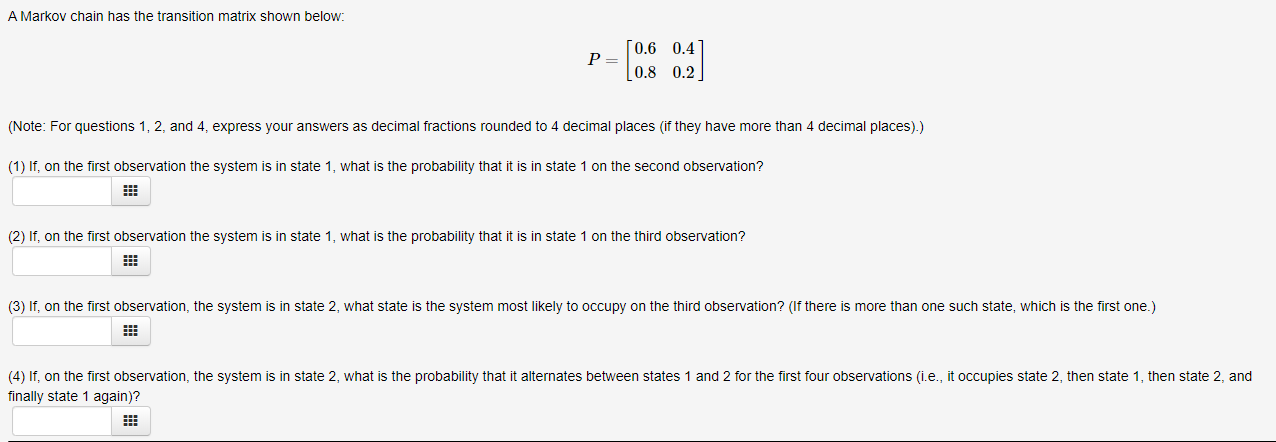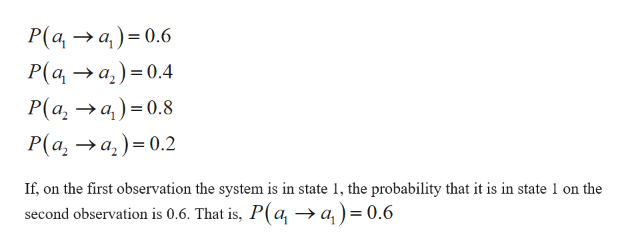# A Markov chain has the transition matrix shown below:[0.6 0.4P =[0.8 0.2](Note: For questions 1, 2, and 4, express your answers as decimal fractions rounded to 4 decimal places (if they have more than 4 decimal places).)(1) If, on the first observation the system is in state 1, what is the probability that it is in state 1 on the second observation?(2) If, on the first observation the system is in state 1, what is the probability that it is in state 1 on the third observation?(3) If, on the first observation, the system is in state 2, what state is the system most likely to occupy on the third observation? (If there is more than one such state, which is the first one.)(4) If, on the first observation, the system is in state 2, what is the probability that it alternates between states 1 and 2 for the first four observations (i.e., it occupies state 2, then state 1, then state 2, andfinally state 1 again)?

Question
33 viewshelp_outlineImage TranscriptioncloseA Markov chain has the transition matrix shown below: [0.6 0.4 P = [0.8 0.2] (Note: For questions 1, 2, and 4, express your answers as decimal fractions rounded to 4 decimal places (if they have more than 4 decimal places).) (1) If, on the first observation the system is in state 1, what is the probability that it is in state 1 on the second observation? (2) If, on the first observation the system is in state 1, what is the probability that it is in state 1 on the third observation? (3) If, on the first observation, the system is in state 2, what state is the system most likely to occupy on the third observation? (If there is more than one such state, which is the first one.) (4) If, on the first observation, the system is in state 2, what is the probability that it alternates between states 1 and 2 for the first four observations (i.e., it occupies state 2, then state 1, then state 2, and finally state 1 again)? fullscreen
check_circle

Step 1

1.

The given transition probability matrix can be represented as,

Step 2

The probability of transition for one state to another is,help_outlineImage TranscriptioncloseP(a, → a,) = 0.6 P(a, → a,) =0.4 P(a, → a,) = 0.8 P(a, →a, )= 0.2 If, on the first observation the system is in state 1, the probability that it is in state 1 on the second observation is 0.6. That is, P(a, → a,)= 0.6 %3D fullscreen
Step 3

2.

If, on the first observation the system is in state 1, the probab...

### Want to see the full answer?

See Solution

#### Want to see this answer and more?

Solutions are written by subject experts who are available 24/7. Questions are typically answered within 1 hour.*

See Solution
*Response times may vary by subject and question.
Tagged in
MathStatistics